# Modeling A Superconducting Fault Current Limiter Inserted in a Nine-Bus Electrical Network

Modeling A Superconducting Fault Current Limiter Inserted in a Nine-Bus Electrical Network
Department of Electrical Engineering, Faculty of technology, University of M’Sila, 28000, Algeria

Corresponding Author Email:
salah.belkhiri@univ-msila.dz
Page:
73-78
|
DOI:
https://doi.org/10.18280/mmc_a.922-406
2 February 2019
|
Accepted:
4 May 2019
|
Published:
30 December 2019
| Citation

OPEN ACCESS

Abstract:

Industrial electrotechnics represents a considerable potential market for superconducting materials. Among the feasible applications, the limitation of the fault current will probably be the first to find an industrial departure. We present in this work the results of the simulations of the magnetic and thermal behavior of a fault current limiter designed from a high critical temperature superconducting layer. The results are obtained from a 3D computation code, developed and implemented under MATLAB environment where the formulation in magnetic vector potentials A and electrical scalar potential V are adopted to solve the electromagnetic problem and the heat diffusion formulation is also adopted to solve the thermal problem by the method of the finite volumes. The results showed the interest of designing a superconducting fault current limiter. The development of a simulation model that describes the behavior of the (SFCL) has also been implemented in a nine-bus network.

Keywords:

finite volume method, superconducting faults current limiter, short circuit, bus, electromagnetic and thermal phenomena

1. Introduction

The rapid limitation of fault currents remains an unsolved problem in power grids and electrical, mechanical and thermal damage is still unavoidable. The use of fault current limiters designed from high temperature critical superconducting materials (SFCL) has made it possible to limit fault currents in power grids [1, 2]. In the event of a short-circuit, the latter must not only be able to withstand this fault regime and act as natural current regulators, but also reduce the mechanical and thermal stresses experienced by the network .

The characteristics involved are quite specific to superconductors, namely the great difference existing between a passing state with high current density, and a blocking state with high resistivity, obtained as soon as the current exceeds a given threshold. It is thus possible to protect the electrical network against any rise in the current beyond a specified value. These limiters offer the advantage on the one hand, to be invisible in the rated or rated speed and to be able to limit the fault currents in a very short response time (under 05 ms) compared to conventional current limiters or conventional circuit breakers .

These advantages, which are specifically offered by current limiters designed from high critical temperature superconductors, have led to their insertions with great success in medium and high voltage power grids . To ensure an excellent insertion of the superconducting current limiter, in particular during the fault regime within the electrical network, it is essential to study its magnetic and thermal behavior in order to avoid certain problems that can damage the superconducting material after each limitation. Such as, a significant rise in temperature within the superconductor which is due to a large and rapid increase of the fault current during the process of limitation .

It is not possible during a test to try all the possible configurations of short-circuit on a network, according to the type of network (overhead or underground), according to the impedance of the fault, and according to the power of the network. It is therefore interesting to have modeling tools to simulate the behavior of a superconducting current limiter (SFCL) and to extrapolate the results obtained to other short-circuit configurations and other voltage levels network [7, 8]. The coupling is ensured by an alternating algorithm and the numerical resolution of the problem is ensured by the code implemented under MATLAB environment in order to avoid certain problems of numerical convergence linked to the strongly nonlinear character of the problem to be solved.

2. Modeling Electromagnetic Phenomena

In this paper, we present the modeling of the magneto-thermal behavior of superconducting materials used in the field of electrical engineering precisely the electrical networks. The magneto-thermal phenomenon presented by these materials corresponds to a strong coupling between the magnetic and thermal properties. In this context, several simulation works have been proposed. In some of these works, the behavior of the superconductor is simulated as a vary-resistance [4, 9] where the superconducting material changes from non-dissipative state characterized by zero resistance in the rated regime of the network to a non-dissipative state. Very dissipative state characterized by a high resistance in the case of faults that can appear during the operation of the electrical network. These simple models developed do not satisfactorily reflect the actual behavior of the superconductor in its intermediate state, particularly the FLUX-FLOW and FLUX CREEP regimes. For this, other microscopic models have been proposed in order to satisfactorily describe the FLUX-FLOW and FLUX CREEP regimes [9, 10]. In these models, Maxwell's equations are adopted and coupled to the heat diffusion equation. The critical state model states that at given temperature the current density in a superconductor is either zero or equal to the critical current density Jc.

From a mathematical point of view, in Maxwell's equations, this translates into:

$\overrightarrow{r o t}(\vec{E})=-\frac{\partial \vec{B}}{\partial t}, \operatorname{div}(\vec{B})=0, \overrightarrow{\operatorname{rot}}(\vec{H})=\vec{J}$       (1)

As with other drivers, the following equations complete Maxwell's equations:

$\vec{B}=\mu(\vec{H}), \quad \vec{J}=\sigma \vec{E}, \quad \vec{E}=\rho \vec{J}$            (2)

-$\vec{E}$ is the electric field (in V ${m}^{-1}$ ), $\vec{B}$ is the magnetic induction field (in T).

-$\vec{H}$ is the magnetic field (in A m-1), $\vec{J}$ is the electrical current density (in A m-2).

-$\sigma$ is the conductivity of the medium (in $\Omega^{-1}$ m-1), ρ is the resistivity of the medium (in $\Omega$ m).

-$\mu$ is the magnetic permeability of the medium (in H m-1).

The Bean model assumes, in addition, that the critical current density is independent of the value of magnetic induction B. This model has the advantage of being fairly simple mathematically and allows for simple geometries to have Analytical expressions and study important quantities for AC losses for example. However, the discontinuity of this model renders it useless for numerical developments; moreover, it does not always satisfactorily reflect the behavior of the superconductors because, in reality, the current density strongly depends on its field orientation and magnetic induction, B. An expression of Jc (B) in the isotropic case was given by Kim's model.

$J_{c}(|B|)=\frac{J_{c 0} B_{0}}{|B|+B_{0}}$       (3)

The power model that models well the behavior of high-temperature superconductors (HTC) around Jc. The variation parameters of this law are the critical current density, Jc and the exponent, "n". With this model we can vary the curves E (J) so that we can model a normal conductor for n=1 (linear behavior law) until a steep curve is obtained, as in the case of the critical state model for n> 100 [3, 11].

$E=E_{c}\left(\frac{J}{J_{c}}\right)^{n}$          (4)

The Flux Flow and Flux Creep model has made it possible to define two modes of operation for the superconductor, according to the value of the critical current density Jc:

• If  $|\mathrm{J}| \leq \mathrm{Jc}$, the vortex network is anchored, however, vortices move from one anchor site to another under the action of thermal agitation. This dissipative phenomenon is called the "Creep flux" regime.

$E=2 \rho_{c} J_{c} \sinh \left(\frac{U_{0} J}{k \theta J_{c}}\right) \exp \left(-\frac{U_{0}}{k \theta}\right)$           (5)

K: Constant of Boltzmann, θ: Temperature, ρc: Resistivity of Flux Creep, U0: Potential of depth.

• If  $|\mathrm{J}|>\mathrm{J}_{\mathrm{C}}$, the vortex network moves and generates losses showing an electrical resistance in the superconducting material. This phenomenon is called the "flow flux" regime.

$E=\pm\left(E_{C}+\rho_{f} \cdot J_{c}\left(\frac{|J|}{J_{C}}-1\right)\right)$               (6)

ρf: Resistivity of Flux Flow,

The critical current density can then be defined as the boundary between the creep flow regime and the flow flux regime. Since this limit is very fuzzy, the critical current density is often determined by the value of a critical electric field Ec. The variable resistance model is founded when the current becomes abnormally high , the transition from the superconductive state to a highly dissipative state allows the insertion of an impedance in an electric circuit and therefore the limitation of the current (Rsc goes from zero in the regime assigned to a maximum value during the fault). The determination of Rsc max depends essentially on the nature of the superconducting material used in the design of SFCL (Bi2223 or YBCO) and the design of the electrical network [13, 14].

## 1.png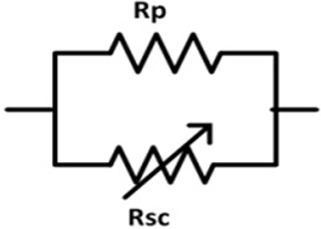Figure 1. Model with variable resistance

## 2.png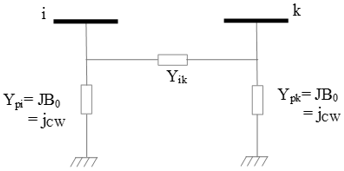Figure 2. Equivalent diagram of a transmission line

where,

$\operatorname{Rsc}=\left\{\begin{array}{lr}o & (J<J c, T<T c) \\ \operatorname{Rc}\left(\frac{J}{J c}\right)^{n-1} & \text { if }(J>J c, T<T c) \\ \operatorname{Rsc} \max (T) & \quad(T>T c)\end{array}\right.$             (7)

Rsc: superconducting resistance, Rc: critical resistance of the superconductor,

Rsc max: Max superconducting resistance after the transition,

## 3.png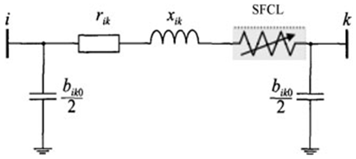Figure 3. Model of SFCL inserted in the transmission line

## 4.pngFigure 4. Model of SFCL inserted in series with generator

The equivalent circuit in π of the transmission line with SFCL is illustrated in Figure 3. The following admittance matrix of the line can be formed :

$Y_{i k}=\frac{1}{\left(r_{i k}+R_{S F C L}\right)+j x_{i k}}$                 (8)

where, rik, xik, and bikare resistance, reactance line, and capacitance line, respectively. RSFCL is resistance of SFCL.

The equivalent circuit of generator equipped by SFCL is given by the figure 4.

When the resistive SFCL is placed in series with generator, the element of the admittance matrix is given by

$Y_{i k}=\frac{1}{\left(R_{G}+R_{S F C L}+J X_{d}^{1}\right)}$             (9)

where, $X_{d}^{1}$ is the d-axis generators transient reactance of generator, RG is resistance of generator. RSFCL are resistance of resistive SFCL, respectively.

So after inserting the SFCL into the network, there will be a significant change in the admittance matrix.

We present the simulation results obtained during a fault on a power grid with and without a current limiter using the PSIM software. The computation of Rsc max is done from the numerical code developed and implemented under the MATLAB environment, where we adopted the method of the finite volumes as method of resolution of the set of partial differential equations characteristic to the physical phenomena to be treated.

3. Presentation of Simulation Parameters

The basic principle of the current limiter involves the properties of YBaCuO which vary considerably depending on the temperature. YBaCuO is a superconductor having a critical temperature TC of the order of 92 K.

This part sets up the choices made on the materials and properties that will be used for the simulations that will follow. Figure.5 shows the crystallographic structure of YBaCuO.

## 5.png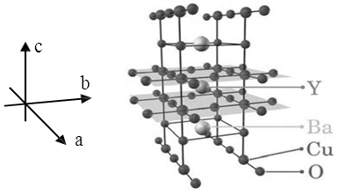Figure 5. Crystallographic structure of YBaCuO [11-12]

The following table gathers the geometrical parameters and characteristics of the material studied YBCO by our simulation code.

Table 1. Parameters of the simulation for the YBaCuO pellet

 Symbol Amount Value Tc Critical temperature 92 K Ec Critical electric field 1×10-4 V/m Jc Critical current density 5×107 A/m2 n0 Exponent n at 77 K under zero field 20 SFCL Superconducting fault current limiter (Lx, Ly, Lz)= (4×4×4) mm3
4. Application and Results

4.1 Spatial distribution of temperature t within the superconducting pellet (SFCL)

The results below represent the three-dimensional distribution of the temperature within the superconducting pellet used to limit the fault current. The temperature within the pellet increases gradually over time, which is to say with the increase of the fault current. The temperature reaches its maximum at the heart of the superconducting pellet and it decreases considerably on the walls of the pellet, this is due to the cooling effect of the cryogenic fluid.

## 6.png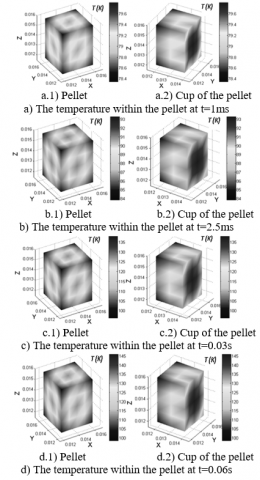Figure 6. Spatial distribution of the temperature within the pellet

When the current exceeds the critical current, the transition is initiated. The energy dissipated at the pellet heats the limiter constantly and homogeneously. If the pellet heats up to its critical temperature Tc, the material then passes by exceeding its critical temperature and goes into its normal state (transition from the superconducting state to the normal state).

## 7.png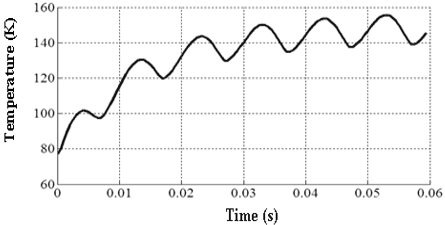Figure 7. The variation of the temperature in the pellet as a function of time

Figure 6.a. Corresponds to the superconducting state of the pellet, the result has been noted at time t=1ms and in this case the limiter no longer intervenes. Because the maximum temperature inside the pellet is of the order of 79.8 ° K is lower than Tc.

Figure 6.b, c and d. Respectively recorded at times t=2.5 ms, t=0.03 s and t=0.06 s show how the limiter takes its normal state and comes into play limitation, the temperatures in the pellet are excessively of the order of 93.3 °K, 137.8 °K and 145.6 °K.

## 8.png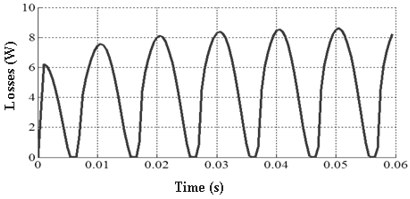Figure 8. The variation of the average losses in the pellet as a function of time

Figure 7 Clearly shows the temporal evolution of the temperature within the superconducting pellet. It appears a beginning of transition at time t=2.5 ms, corresponds to a temperature close to the critical temperature.

Figure 8 illustrates the temporal evolution of the average losses in the pellet. These are increased over time because of the energy dissipated per unit volume within the superconducting pellet.

4.2 Inserting the SFCL into the network at nine- bus

4.2.1 Case of a two-phase fault

## 9.png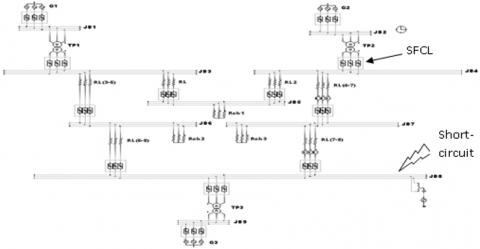Figure 9. Nine-bus network with two-phase fault with SFCL

A short-circuit two-phase at the bus 8. PHASES terminals (V-W) is applied at time t=0.1s and for good visibility, the simulation is performed over a period of 0.4 s.

(1) Currents of the Line (4-7):

## 10.png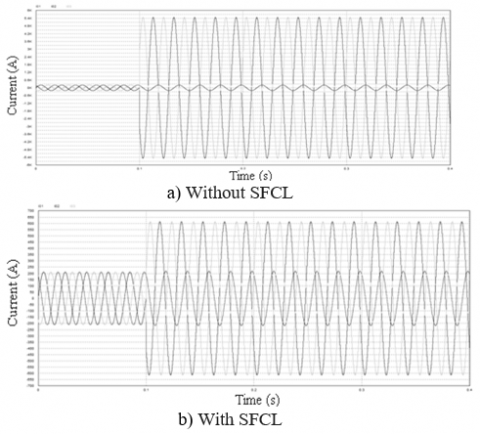Figure 10. The pace of currents I1, I2, I3 of the line (4-7)

(2) Currents of the line (7-8):

## 11.png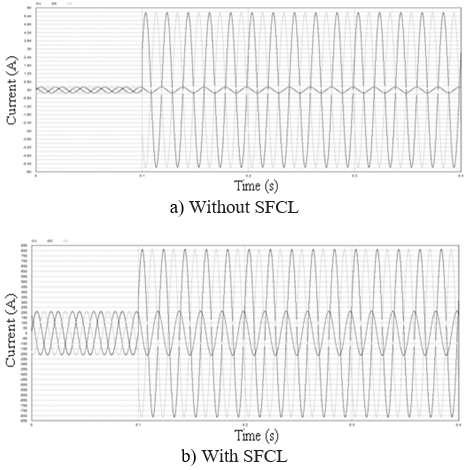Figure 11. The pace of the currents I4, I5, I6 of the line (7-8)

(3) Voltages of the line (7-8):

## 12.png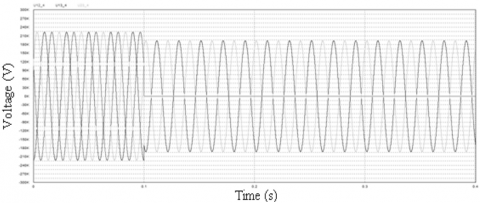Figure 12. Waveform of the voltages U12, U23, U13 of the line (7-8) during two-phase fault

(4) Voltages of the line (4-7):

## 13.png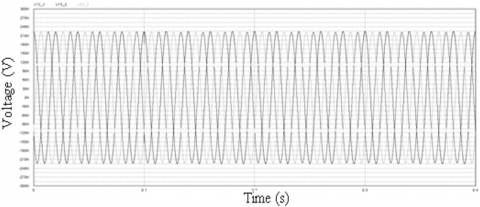Figure 13. Waveform of the voltages U12, U23, U13 of the line (4-7) during two-phase fault

The Table 2 presents and collates the results of the currents recorded in the line (4-7) and in the line (7-8), also the transition time of SFCL.

Table 2. Two-phase short-circuit current values with and without SFCL limitation

 Line Default in the Bus Rated current In (A) Icc without SFCL (A) IL=ICC with SFCL (A) Transition time of SFCL (ms) 4-7 8 204 5470 ≈ 27 In 613 ≈ 3 In 4 7-8 8 204 5650 ≈ 28 In 814≈ 4 In 4

4.2.2 Case of a three-phase fault

In this case, a short-circuit three-phase in the bus 8, phases terminals (U-V-W) is applied at time t=0.1s. The Table 3 presents and collates the results of the currents recorded in the line (4-7) and in the line (7-8), also the transition time of SFCL.

Table 3. Values of three-phase short-circuit currents with and without SFCL limitation

 Line Default in the Bus Rated current In(A) Icc without SFCL (A) IL=ICC  with SFCL (A) Transition time of  SFCL (ms) 4-7 8 204 6310 ≈ 31 In 613≈ 3 In 4.5 7-8 8 204 6520 ≈ 32 In 814≈ 4 In 4.5

Figure 14 shows the voltages per phase of the line (7-8), whenever SFCL is placed in the power system network, precisely in bus 8.

## 14.png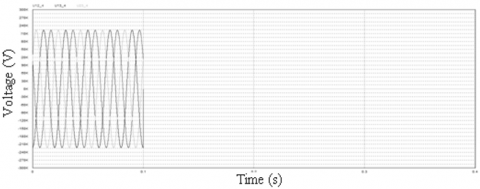Figure 14. Waveform of the voltages U12, U23, U13 of the line (7-8) during three-phase fault

5. Interpretation and Discussion

The results show the two-phase and three-phase current waveforms in the simulation with and without SFCL when a fault occurs, it could be noted that when there is no SFCL inserted in the nine-bus network, the peak value of the fault current could be as high as about 6.5KA. However, when with SFCL, the same system would have a peak fault current of only about 814A. Therefore, the SFCL effectively limits the peak value of the fault current as shown in Table 2 and 3.

As shown in Figure 11, the nominal current of the line (4-7) is In=204 A, until the fault is applied at t=0.1 s from this instant. The fault current Icc=5.47KA (Icc=27In) for the two defective phases (VW), otherwise phase (V) remains healthy with a current of 204 A which is the nominal value. After inserting the limiter it is clear that the current is limited from a value of 5.47 kA without SFCL to a value IL=613 A, with SFCL at the moment of application of the fault (0.1s) where the fault current exceeds the critical current, therefore the intervention of the current limiter where it plays the role of a high-value resistor (the transition of the superconductor from the state to the purely resistive state).

In the Figure 12 the nominal current of the line (7-8) is In=204 A, until the fault is applied at t=0.1 s from this instant. The fault current Icc=5.65 kA (Icc=28In) for of the two defective phases (VW), moreover the phase (U) remains healthy with a current of 204 A which is the nominal value and for the voltages it is seen that a crushing of the voltage U12 due to the grounding of the two phases V and W. After inserting the limiter, it is clearly seen that the current is limited from a value of In=5.65 kA without SFCL to a value IL=814 A at the moment of application of the fault (0.1s).

The comparison of the results obtained for the two types of two-phase and three-phase short-circuit, figures 13 and 14 shows that the transition time and the limited current (IL) by the SFCL are practically the same.

A 3-phase fault on line (7–8) the voltages U12, U23, U13 we see that a crushing (its value equal to zero) of the three voltages because of the grounding of the three-phase U, V and W.

6. Conclusion

Using coupled electromagnetic magnitudes in superconductors, a numerical model was constructed. An expression of the variation of the SFCL impedance due to the presence of the short-circuit current has also been established while explaining the geometric and electromagnetic characteristics of the SFCL (modification of the matrix admittance of the system). In order to simulation an SFCL, it is necessary to understand the transition from the superconducting state to the normal state. The simulation results obtained are very satisfactory.

References

 Rao, M.U.M., Rosalina, K.M. (2018). Microgrid protection by using resistive type superconducting fault current limiter. Modelling, Measurement, and Control A, 91(2): 89-93. Microgrid protection by using resistive type superconducting fault current limiter

 Sarkar, D., Roy, D., Choudhury, A.B., Yamada, S. (2016). Harmonic analysis of a satured iron- core superconducting fault current limiter using Jiles-Ather hysteresis model. AMSE JOURNALS-2016-Series: Modelling A, 89(1): 101-117.

 Cointe, Y., Doctoral thesis Continuous. (2007). Superconductive Limiter. Engineering Sciences [physics]. National Polytechnic Institute of Grenoble - INPG, 2007. French. <Tel-00300552>

 Saad, N. (2013). Modelling and simulation of the superconducting current limiter. Doctoral thesis, Sétif University, Algeria.

 Kado, H., Ickikawa, M. (1997). Performance of a high-Tc superconducting fault current limiter-design of a 6.6 kV magnetic shielding type superconducting fault current limiter. IEEE Transactions on Applied Superconductivity, 7(2): 993-996. https://doi.org/10.1109/77.614672

 Chan, W.K., Masson, P.J., Luongo, C., Schwartz, J. (2010). Three-dimensional micrometer-scale modeling of quenching in high-aspect-ratio YBa2Cu3O7−δ Coated conductor tapes—Part I: Model development and validation. IEEE Transactions on Applied Superconductivity, 20(6): 2370-2380. https://doi.org/10.1109/TASC.2010.2072956

 Leonidopoulos, G. (2015). Modelling and simulation of electric power transmission line voltage. AMSE JOURNALS-2016-Series: Modelling A, 88(1): 71-83.

 Leonidopoulos, G. (2016). Modelling and simulation of electric power transmission line current as wave. AMSE JOURNALS-2016-Series: Modelling A, 89(1): 1-12.

 Yamaguchi, H., Kataoka, T. (2007). Current limiting characteristics of transformer type superconducting fault current limiter with shunt impedance. IEEE Transactions on Applied Superconductivity, 17(2): 1919-1922. https://doi.org/10.1109/TASC.2007.898494

 Chan, W. K., & Schwartz, J. (2011). Three-dimensional micrometer-scale modeling of quenching in high-aspect-ratio YBa2Cu3O7−δ coated conductor tapes—Part II: Influence of geometric and material properties and implications for conductor engineering and magnet design. IEEE Transactions on Applied superconductivity, 21(6), 3628-3634. https://doi.org/10.1109/TASC.2011.2169670

 Mahdad, B., Srairi, K. (2013). Application of a combined superconducting fault current limiter and STATCOM to enhancement of power system transient stability. Physica C: Superconductivity, 495: 160-168. https://doi.org/10.1016/j.physc.2013.08.009

 Zhu, J., Zheng, X., Qiu, M., Zhang, Z., Li, J., Yuan, W. (2015). Application simulation of a resistive type superconducting fault current limiter (SFCL) in a transmission and wind power system. Energy Procedia, 75: 716-721. https://doi.org/10.1016/j.egypro.2015.07.498

 Alloui, L., Bouillault, F., Bernard, L., Leveque, J., Mimoune, S. M. (2012). 3D modeling of forces between magnet and HTS in a levitation system using new approach of the control volume method based on an unstructured grid. Physica C: Superconductivity, 475: 32-37. https://doi.org/10.1016/j.physc.2012.01.012

 Alloui, L., Bouillault, F., Mimoune, S. M. (2009). Numerical study of the influence of flux creep and of thermal effect on dynamic behaviour of magnetic levitation systems with a high-T c superconductor using control volume method. The European Physical Journal-Applied Physics, 45(2): 191-195. https://doi.org/10.1051/epjap/2009008